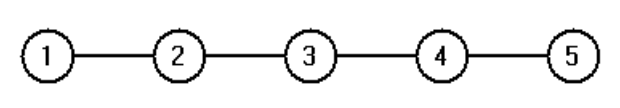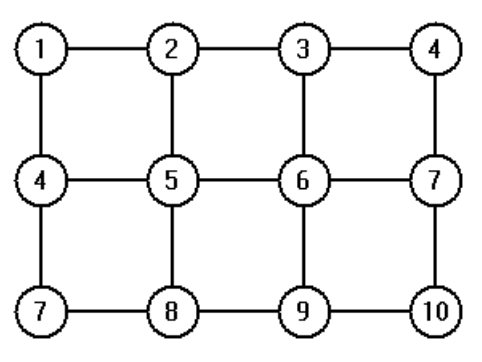시간 제한 메모리 제한 제출 정답 맞은 사람 정답 비율
1 초 512 MB 0 0 0 0.000%

## 문제

In the Pass the Buck game, there are several players and each player has one or more neighbors among the other players.

Each game begins with one player chosen at random who receives a buck (the current holder). (For this game, chosen at random means each possible outcome has the same probability.)

Then, at each step, if the current holder has d neighbors, he/she chooses an integer k in the range [0, d ] at random. If 0 is chosen, the current holder is the winner and keeps the buck. Otherwise, the holder passes the buck to the neighbor with index k, who becomes the new holder.

The game continues until some holder wins.

Write a program to find the probability that player j wins if player k is the first holder.

The configuration of players and neighbors can be modeled as a graph with the players as vertices and an edge between two vertices if the corresponding players are neighbors. For example, if they are seated in a row then the neighbors are the players to the left and right (if any).If they are seated around a table, then each player has a neighbor to the right and left.

If they are seated in multiple rows, they may have neighbors left, right, in front and/or behind.## 입력

The input consists of multiple lines of input. The first line contains the number, N, (2 <= N <= 20) of players followed by a space followed by the number, P, (1 <= P <= 20) of start/winner pairs.

The next N lines of input give the neighbors of each player. Line m gives the neighbors of player m. The first integer on the line is the number, d(m), of neighbors of player m. This is followed by d(m) integers giving the indices of the neighbors of m, separated by spaces.

The next P lines of input give the index, s, of the start holder and the index, w, of the player whose probability of winning when s is the first holder is to be found. Each line contains three integers, j, s(j) and w(j) separated by spaces where j is the index of the pair.

## 출력

Your program will generate P lines of output.

The jth line contains the integer j a space and a 5 decimal point value which is the probability that player w(j) wins if player s(j) is the first holder.

## 예제 입력 1

5 4
1 2
2 1 3
2 2 4
2 3 5
1 4
1 1 1
2 2 2
3 3 3
4 4 4


## 예제 출력 1

1 0.61818
2 0.47273
3 0.45455
4 0.47273